Thursday, 15 March 2018

Triangle area formulae involving the inradius and circumradius

While laid up over the last few weeks following surgery, I wrote an article containing some ideas that try to convey clearly how interesting (and comprehensible) these formulae are. I'm not breaking any new mathematical ground here, of course, but I was interested in how well I could illustrate the ideas with diagrams. You be the judge... (rather than try cram the whole thing into a blog post, I've just outlined the main ideas below. Please for all the details).

The first diagram shows the "base triangle" ABC, with sides labelled a, b and c in the conventional way.

This diagram shows the inscribed circle or incircle of triangle ABC, with radius r (the inradius of the triangle). This is the largest circle that can be drawn inside the trangle and is tangent to all three sides. The formula makes use of the semiperimeter, s = (a + b + c)/2.

The area of the triangle is simply rs. The article contains a couple of different proofs of this.

This diagram shows the circumscribed circle or circumcircle of triangle ABC, with radius R (the circumradius of the triangle). This is the smallest circle that completely contains the triangle, passing through all three vertices.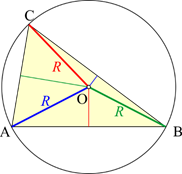The area of the triangle is abc/4R. The article contains a proof, but also looks at the link between the circumradius and the sine rule and how this could possibly help to demystify why the rule works.

Feel free to leave comments!

Kinsler Crosses

In his excellent book A Square Peg in a Round Hole: Adventures in the Mathematics of Area, Dr Chris Pritchard introduces an idea he calls ‘Kinsler’s Cross’ after the colleague who introduced it to him.
This article provides a detailed walkthrough of how Kinsler crosses can be applied to find the area of a triangle and thereby justify the traditional formula A = ½bh.
The cross ‘works’ as follows: inside the figure whose area is to be calculated, draw two perpendicular line segments, as shown in this diamond:

The idea is to find positions for the line segments such that their product is equal to the area of the figure: in other words, such that the area of the yellow product rectangle below is equal to the area of the diamond:
This obviously isn’t the right position in the case shown above: there’s more of the rectangle outside the diamond than there is unfilled area within it.

Crosses in triangles

For a triangle, you need to drop a perpendicular from one vertex to the opposite side (one of the triangle’s altitudes) to form one segment of the cross:

Here are a few possible positions for the second segment, with the product rectangles shown:

The first clearly gives an area that is too small, while the second is too large. It turns out that when the second segment joins the midpoints M and N of the two sides it touches, the area is correct.

This is easy to demonstrate by dissecting the rectangle and rotating some of the parts. First, split the rectangle into three parts as shown below:

Part A is completely inside the triangle, so it does not need to be moved. To show it has become fixed, I’ve coloured it pink. The two ‘exterior’ parts of the rectangle are now labelled B and C:

Next, rotate B through a half-turn about midpoint M:

Now rotate C about N in the same way:

The whole of the rectangle is now contained within the triangle.

Now, the second segment of the cross joins the midpoints of two sides of the triangle:

The smaller triangle is similar to the original and half the size, meaning that MN is half the base of the original triangle. The area of the product rectangle is thus ‘half the base’ times ‘the height’.

The mathematical meaning of A = ½bh is usually taken as ‘half of (the base × the height)’, which is justified by doubling the triangle to make a rectangle. To remind yourself how this works, use the altitude that formed the first segment of the cross to split a copy of the original triangle into two right triangles, as shown here:
Now rotate both of the right triangles through a half-turn:
Finally, match up the hypotenuses of the right triangles with the corresponding sides of the original triangle:

This forms a rectangle whose area is twice that of the original triangle.
The base of this rectangle is equal to the base of the triangle, and it has the same height as the triangle. Hence, ‘half of (the base × the height)’.
However, students will often quote the formula as ‘half the base’ times ‘the height’, and will even calculate with it in this sense. The Kinsler cross provides an appropriate justification for this perfectly reasonable parsing of A = ½bh.

Obtuse triangles

The Kinsler cross method can be used in any obtuse triangle in the straightforward manner illustrated above, by choosing the longest side of the triangle as base (this is the one opposite the obtuse angle, by Proposition I.19 of Euclid’s Elements). However, it’s also possible to demonstrate how the process works using the ‘horizontal’ base, as follows. The dissection is more complicated, but the result is very satisfying!

The rule that the altitude has to pass through a vertex makes it impossible to see a cross at all, let alone a product rectangle; however, if I translate the altitude to the midpoint of the second segment, the situation becomes more obvious. Does it look as if the rectangle has the same area as the triangle?

The process starts by identifying that part of the rectangle that is currently within the triangle. This is the trapezium marked A below. Then the protruding portions of the rectangle are labelled B and C (they are both right-angled trapezia). The midpoints are labelled M and N, as before.

Next, rotate B and C through half-turns about N and M respectively:

The parts of B and C that now lie within the triangle are labelled D and F, respectively. This leaves two protruding parts: the right triangle E and trapezium G.
Now rotate E and G by half-turns about M and N, respectively:

G is now entirely contained within the triangle; E needs to be split into triangle H, which is inside the triangle, and right triangle I, which is outside.
Finally, rotate I through a half-turn about N:
All of the parts made by dissecting the rectangle are now inside the triangle. Just to show that the rectangle really can be recreated from the pieces, the second diagram below shows what they look like when reassembled:
This configuration of the constituent parts yields some interesting relationships. In the diagram below, line segments that are parallel to the sides of the triangle have been identified. Note the intersections of the produced segments with the lower vertices of the triangle. Also, segments that are parallel to each other are necessarily of the same length.
The number of times this dissection/rotation process has to be carried out will depend on the ratio of height to base of the rectangle, and also the size of the obtuse angle. To analyse this, it will be easier to think of the triangle being dissected to form the rectangle, rather than the other way around.
In the diagram above, the number of strips n into which the triangle will have to be divided to form a rectangle of width ½b will be (x + b) divided by ½b, rounded up to give a whole number, as there is no reason why this should be an integer. Note that the following lines use the ceiling function: the notation
denotes the smallest integer greater than or equal to a.

In the right triangle with base x,
Therefore
As a check, in the example used above to illustrate the process, b = 2, h = 6 and tan θ = -3/2.

The diagram confirms this, each of the parts H, F, A, D, G and I constituting one strip.

References

Densmore, D (ed., 2002). Euclid’s Elements: all thirteen books complete in one volume: the Thomas L. Heath translation. Santa Fe: Green Lion Press.
Pritchard, C (2016): A Square Peg in a Round Hole: Adventures in the Mathematics of Area. Leicester: The Mathematical Association.

Thursday, 21 July 2016

Going Loopy

One the things I've been fooling around with this year is Polygon Loops. Anyone remember the old Octagon Loops task from the early days of the National Curriculum, with its translucent green stencils?Loop of eight regular octagons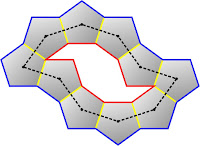Loop of ten regular pentagons
This task generalises nicely to other types of regular polygons (and combinations of regular polygons) and there is some heavyweight literature on it, too (see the references below).

References
Griffiths, M (2001): Two proofs concerning `Octagon loops', Mathematical Gazette 85, 80-84.
McLean, K R (2000): Loops of regular polygons, American Mathematics Monthly 107, 500-510.

Thursday, 27 August 2015

Progressions in PolygonsYou know how it is. You start tinkering around with an idea and before you know it, it's a month later and you've written 40 pages' worth of stuff. That's what happened when I started messing around with patterns in the interior angles of polygons this summer. The picture above is of an octagram whose angles form an arithmetic progression.

I started out by looking at simple alternating angle patterns, then moved onto APs and GPs, and finally quadratic sequences. It's not an exhaustive piece of work by any means, but there are lots of interesting discoveries in there. For example, the AP relationships in a quadrilateral (illustrated below) force any such quadrilaterals either to be cyclic, or trapezia! I love it when this kind of unexpected simplicity pops out of what appears to be a complex investigation.
There are lots of places to go with this, too... specific sequences such as polygonal numbers;  into three dimensions with angular deficiency or skew polygons, etc. It's not difficult mathematics, but I suspect I may have poked round in the odd unexplored corner, even if it's only because nobody else could be bothered!

Mathematical Idea Analysis

I was switched onto George Lakoff and Rafael Núñez' seminal work Where Mathematics Comes From: How the Embodied Mind Brings Mathematics into Being this summer. Understanding it was quite difficult for someone like me who's not a cognitive scientist, but the writing is very clear and the ideas are fascinating. When we finally got all the way to the Euler equation in the fourth case study I felt like applauding!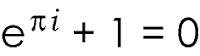I do have some issues with their interpretation of the consequences of the theory as a total refutation of the Platonic position (regarding the independent existence of mathematical objects), but that doesn't invalidate what they say regarding the cognitive structures behind mathematical thought.

However, I'm looking forward to sharing some of this thinking with this year's cohort of trainees. It's always good to look at the "nuts and bolts" of our subject, but here we'll be investigating how the nuts and bolts themselves are made!

Monday, 12 March 2012

Royal Institution Maths Masterclasses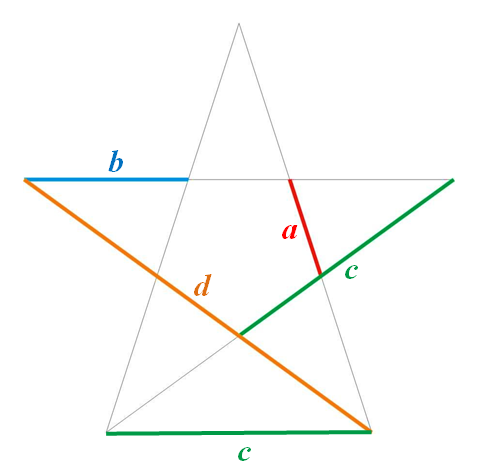Significant lengths in a pentagram...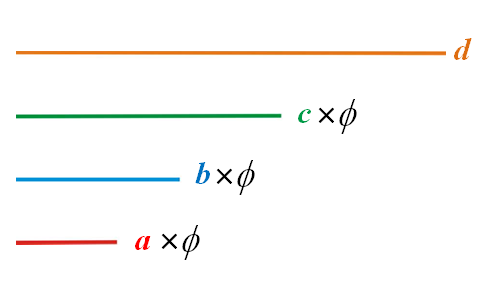...are related by the golden section.

I've been fortunate enough to be involved with these for the last couple of years. The Lincolnshire masterclass series for Year 8 pupils is organized by John Slater, with support from Yvonne Croasdaile of the Further Mathematics Support Programme and Robert Heathcote of Queen Elizabeth's Grammar School, Horncastle.

It was my turn to deliver on Saturday 10th March: my class is called "What Happens Eventually?" and is basically about iterative processes, with refreshment stops at the Golden Section and Stupidly Large Numbers! However, I've been finding that there's a lot of unused material left over, so I think next time I'll do the remainder, once I've checked it will work standalone.

The masterclasses run from 10am until 12.30pm, with a short break; we had just over 50 students in one of the larger maths classrooms at Lincoln Christ's Hospital School. The two-and-a-bit hours seemed to flash past and student engagement seemed high - I'm awaiting feedback!